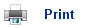# Equilibria and Partial Pressures

• The following reaction between hydrogen and iodine is reversible:

H2(g) + I2(g)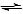2HI(g)

• If it is left for long enough, it will eventually reach dynamic equilibrium; this is where the forward and backward reactions are occurring at the same rate; therefore the concentrations of the reagents do not change.
• The equilibrium constant, Kc, is given by the following expression: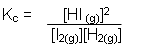• In the equilibrium mixture, it is assumed that each gas behaves independently of the other gases present; the total pressure (P) of the mixture is therefore equal to the sum of the partial pressures of the gases present:

Total Pressure P = PH2 + PI2 + PHI

• The partial pressure of each gas is proportional to its concentration; it is therefore possible to write an expression for the equilibrium constant in terms of partial pressures of the gases: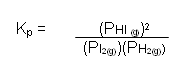• Similarly, it is also possible to write an equilibrium an expression for the equilibrium constant for the reaction between nitrogen and hydrogen to form ammonia:

N2(g) + 3H2(g)2NH3(g)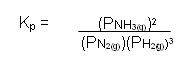• It is much more convenient to write the expression for the equilibrium constant in terms of the partial pressure of the gases.

## Units

• Pressure can be measured in Newtons per square metre (N m-2); this can also be referred to as pascals: 1 Pa = 1 N m-2.
• However, atmospheres (atm) are generally the unit of pressure in equilibrium expressions: 1 atm = 1.01 x 102 kPa.
• Therefore the units for the equilibrium constant for the reaction between iodine and hydrogen can be caluclated: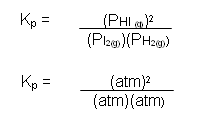• The units cancel out, so there are no units for the equilibrium constant for the reaction between hydrogen and iodine.
• The units for the nitrogen/hydrogen/ammonia equilibrium constant are:• As for Kc, the units for each Kp expression must be worked out.

## Changing the Pressure

• If the total pressure of a gas mixture at equilibrium is increased, then the reaction will respond by reducing the pressure again (Le Chatelier’s principle).
• The pressure exerted by a gas is dependent on the number of gas molecules present; therefore the position of equilibrium will shift to the side with the fewest number of gas molecules if the pressure is increased.
• In the reaction between nitrogen and hydrogen to form ammonia, there are four molecules of gas on the reactant side for every two molecules of gas on the product side:

1N2(g) + 3H2(g)2NH3(g)

• Therefore, if the pressure is increased, the position of equilibrium will shift to the right to oppose the change made to the conditions of the reaction.
• For example, at 1 atm total pressure, the equilibrium % of ammonia is equal to 24%. From this the equilibrium constant can be determined: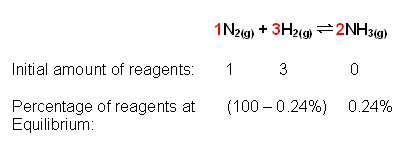The nitrogen and hydrogen react according to a 1:3 ratio, therefore the 99.76% is split into a 1:3 ratio: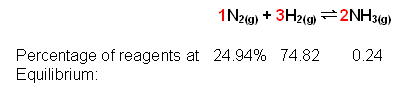Converting these to partial pressures:

PN2 = 0.2494 * 1
PH2 = 0.7482 * 1
PN2 = 0.0024 * 1

Substituting these into the equilibrium expression gives: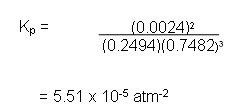• The graph below shows the percentage of ammonia at equilibrium at different pressures: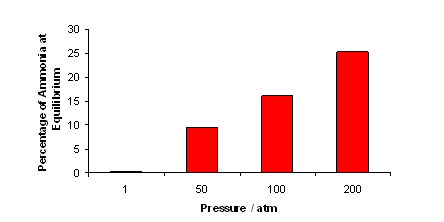• As the pressure increases, the percentage of ammonia at equilibrium also increases; this is why high pressures are used in the Haber process.
• The Kp is constant for each reaction providing the temperature does not change.

## Useful books for revision

Revise A2 Chemistry for Salters (OCR A Level Chemistry B)Salters (OCR) Revise A2 ChemistryHome